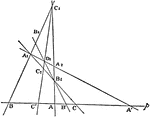### Involution

The three points in which any line cuts the sides of a triangle and the projections, from any point…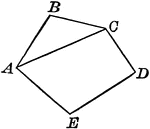### Polygon With Diagonal

Illustration of a polygon with a diagonal drawn. A diagonal of a polygon is a line joining the vertices…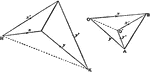### Polyhedron

Orthogonal projection of a closed plane-faced polyhedron.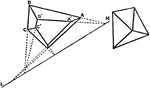### Polyhedron

Orthogonal projection of a closed plane-faced polyhedron.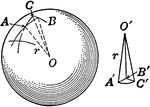### Sphere Cut Into Pyramids.

An illustration of a sphere cut into polygons as bases with their vertices at the center of sphere.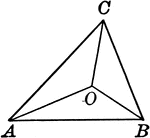### Triangle With Lines From the Vertices That Join a Point Within

Illustration to show lines drawn from the vertices to join at a point within the triangle.